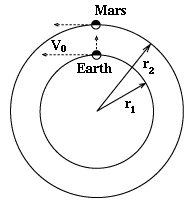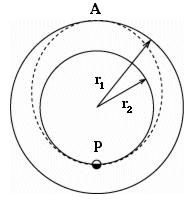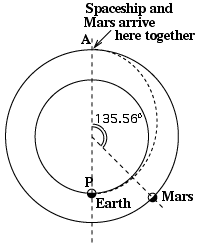# #21b     Flight to Mars: How Long? Along what Path?

 Venus--Hesper--were we native To that splendour or in Mars We should see the globe we groan in Fairest of their evening stars. Could we dream of war and carnage Craft and madness, lust and spite Roaring London, raving Paris In that peaceful point of light? Would we not, when glancing heavenwards On a star so silver-fair Yearn, and clasp the hands, and murmur: "Would to God that we were there"? Alfred, Lord TennysonIndex19.Motion in a Circle20. Newton's Gravity21. Kepler's 3rd Law21a.Applying 3rd Law21b. Fly to Mars! (1)21c. Fly to Mars! (2)21d. Fly to Mars! (3)22.Reference Frames22a.Starlight Aberration22b. Relativity22c. Flight (1)22d. Flight (2)23. Inertial Forces23a. The Centrifugal Force23b. Loop-the-Loop24a.The Rotating Earth24b. Rotating Frames

Using the orbital mechanics developed earlier, we can now plan (with approximations) a space mission to Mars. Assuming our rocket and spaceship are provided--how should they be aimed, and how long would the flight take?

As an approximation assume that the orbits of Earth and Mars are both circles in the same plane, centered on the Sun. The radius of the Earth's orbit, denoted by r1, is close to 150,000,000 kilometers, a distance known as the astronomical unit (AU). That is a convenient unit for measuring distances in the solar system and was used in section #10 on Kepler's Laws. Like there, here too all distances will be measured in AU and all times in years. With such units, for Earth r1 = 1 AU and the orbital period is T1 = 1 year. For Mars (subscript 2), r2 = 1.523691, T2 = 1.8822.

Note: All quantities in this section and in the one following are either non-vectors or magnitudes of vectors. When a quantity is displayed in bold face, this is only done for clear display and carries no connotation of vector character.

### How not to goEarth and Mars at   their closest approach
Those unfamiliar with orbits might think that the best way to reach Mars from Earth is to wait until the two planets are closest to each other (as in the drawing here), then aim the rocket towards Mars and fire away. That is how it was supposedly done in "War of the Worlds" by H.G. Wells, though in that story a giant cannon is used, not a rocket, and the shot goes the other way, from Mars to Earth. That won't work (in either direction) for several reasons.

First, Earth's gravity will bend the trajectory of any spaceship launched from here. To remove that factor assume the rocket has already been placed in a distant orbit around Earth, where Earth's gravity is weak and orbital motion is slow, allowing both to be neglected. In actual orbit planning they must always be taken into account as a correction.

Even then, that rocket is still orbiting the Sun together together with the Earth, to which it is loosely attached, moving at close to 30 km/sec, a velocity we will write as V0. This is much faster than what is needed to reach the Mars orbit (or can be easily provided by rockets!). If we fire when Mars is closest (drawing), V0 is transverse to the aiming direction, so that the spaceship will start in a direction quite different from the one pointing at Mars--and Mars is sure to move away long before it has covered the intervening distance. That is the second reason.

The third reason is that the entire system is dominated by the Sun's gravity. All objects travel in orbits or trajectories, which by Kepler's laws are parts of conic sections--in this cases, ellipses. In general, these are curved.

### Transfer Orbit

So instead of "aim and shoot," it is better search for an orbit which takes the spaceship from Earth to Mars, and to choose to launch when the arrival at the Mars orbit coincides with the arrival of Mars itself at the same location.The Hohmann Transfer Orbit

Mars should be in such a position relative to Earth at the time of launch, that it reaches point A at the same time as the spaceship does. To determine that position one needs to know the duration of the flight from P to A, and that is derived below, using Kepler's 3rd law.

### Kepler's 3rd Law

Kepler's 3rd law as used here has the same form as in Section #10 . For completeness that form is re-derived below. The law states that for all objects orbiting the Sun
T2 / a3 = constant

where T is the orbital period and a the semi-major axis, half the length of the orbital ellipse (a=r in circular orbits). The constant is the same for all objects orbiting the Sun, including of course the Earth. Its exact value depends on the units in which T and a are measured. That value becomes very simple if T is measured in years and a in AU (as is done here). Inserting in the 3rd-law equation the values for Earth gives

T = 1 (year)                     a = 1 (AU)

In our units, then

T2 / a3 = 1

Consequently in these units the constant also equals 1, and that value can be used for any planet. On multiplying both sides of the equation by a3 (second law of algebra)

T2 = a3

This again holds for any orbit around the Sun, including the one of a spaceship in a transfer ellipse. The length PA of that ellipse is, in AU,

r1 + r2   =   1 + 1.523691   =   2.523691 AU

That is the "major axis" of the orbital ellipse, and half its length equals the semi-major axis a. Therefore

a = 1.261845                         a3   =   2.00918   =   T2

Taking the square root

T   =   1.417454 yearsEarth and Mars at launch

That is the time for going from P to A and back to P. The one-way transit time to Mars is half that, i.e.0.70873 years or about 8.5 months.

### Location of Mars

Where should Mars be at the time of launch? From numbers cited at the beginning of this section, it takes Mars 1.8822 years for a full orbit of 3600. Therefore, assuming a circular orbit and uniform motion (a less accurate approximation for Mars than for Earth), in 0.70873 years it should cover

3600 * (0.70886 / 1.88) = 135.5550

We therefore launch when Mars in its orbit is 135.5550 away from point A (drawing)

The next section calculates the velocity which a rocket must impart at point P in order to produce the transfer orbit, and the velocity change required at point A to match velocities with Mars. That calculation is longer and requires some algebra.

Questions from Users:
***
View of Earth and Moon from Mars
***     Fast Trip to Mars     (1)
***     Fast Trip to Mars     (2)
***     Planet Mars "huge in the sky," in August 2005?

Next Stop: #21c. Flight to Mars: The Calculation

Author and Curator:   Dr. David P. Stern
Mail to Dr.Stern:   stargaze("at" symbol)phy6.org .

Last updated: 12-12-2004